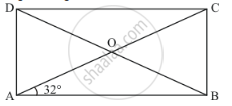Advertisement Remove all ads

# If Abcd is a Rectangle with ∠Bac = 32°, Find the Measure of ∠Dbc. - Mathematics

Answer in Brief

If ABCD is a rectangle with ∠BAC = 32°, find the measure of ∠DBC.

Advertisement Remove all ads

#### Solution

Figure is given as :Suppose the diagonals AC and BD intersect at O.

Since, diagonals of a rectangle are equal and they bisect each other.

Therefore, in ΔOAB, we have

OA = OB

Angles opposite to equal sides are equal.

Therefore,

∠OAB = ∠OBA

∠BAC = ∠DBA

But, ∠BAC = 32°

∠DBA = 32°

Now,

∠ABC = 90°

∠DBA + ∠DBC = 90°

32° +∠DBC = 90°

∠DBC = 58°

Hence, the measure of ∠DBC is 58°.

Is there an error in this question or solution?
Advertisement Remove all ads

#### APPEARS IN

RD Sharma Mathematics for Class 9
Chapter 13 Quadrilaterals
Q 17 | Page 70
Advertisement Remove all ads
Advertisement Remove all ads
Share
Notifications

View all notifications

Forgot password?
Course